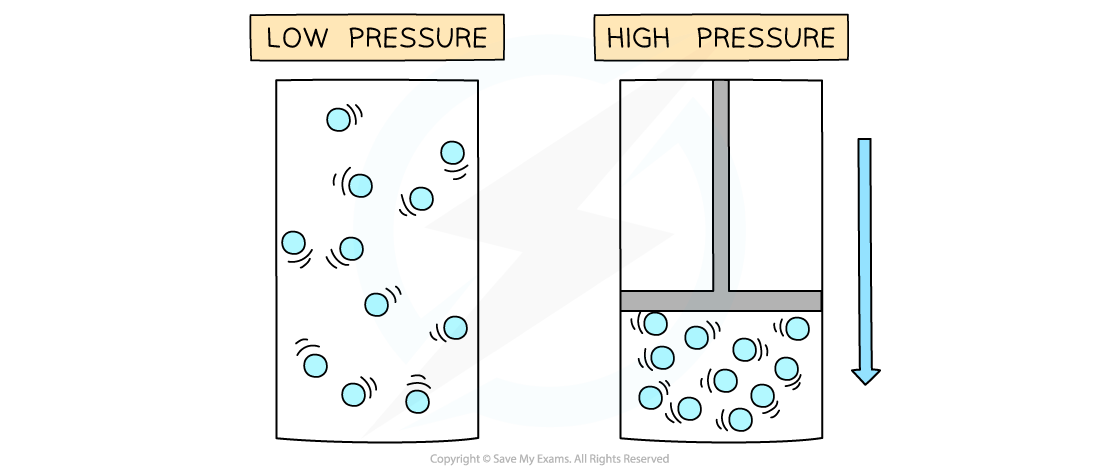# IB DP Chemistry: SL复习笔记1.2.6 Real Gases

### Real Gas Behaviour

• The ideal gas equation does not fit all measurements and observations taken at all conditions with real gases
• The relationship between pressure, volume and temperature shows significant deviation from   PV = nRT when the temperature is very low or the pressure is very high
• This is because the ideal gas equation is built on the kinetic theory of matter
• The kinetic theory of matter makes some key assumptions about the behaviour of gasesAt low temperatures and high pressures real gases deviate significantly from the ideal gas equation. The higher the pressure and the lower the temperature the greater the deviation

• The kinetic theory assumes that the volume the actual gas molecules themselves take up is tiny compared to the volume the gas occupies in a container and can be ignored
• This is broadly true for gases at normal conditions, but becomes increasingly inaccurate at low temperatures and high pressures
• At these conditions the gas molecules are very close together, so the fraction of space taken up by the molecules is substantial compared to the total volumeAt low temperatures and high pressures, the fraction of space taken up by the molecules is substantial

• Another assumption about gases is that when gas molecules are far apart there is very little interaction between the molecules
• As the gas molecules become closer to each other intermolecular forces cause attraction between molecules
• This reduces the number of collisions with the walls of the container
• The pressure is less than expected by the ideal gas equation

#### Exam Tip

The ideal gas equation and the gas constant are given in the IB Chemistry Data Booklet which can be used in Paper 2, but not in Paper 1.